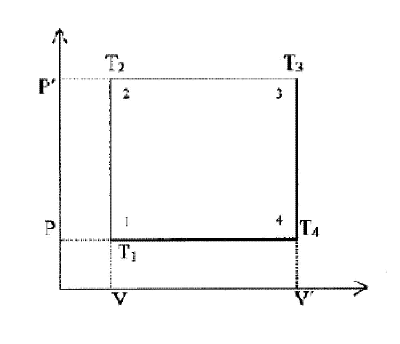## Energy Cycles at Constant Volume

Consider the energy cycle depicted in the P-V diagram below with 2 stages at constant volume and 2 stages at constant pressureInitial state 1 – a fixed mass of gas volume V pressure P and temperature T1

Stage 1-2     the gas is heated at constant volume to temperature T2

raising pressure to P′               Heat input Cv (T2 - T1)

Stage 2-3     the gas is heated at constant pressure to temperature T3

and volume V′                        Heat input Cp (T3 - T2)

Stage 3-4     work done by gas at constant volume so that pressure

falls to P and temperature fall to T4

There is no heat input Q = DU + W = 0

work done by the gas Cv (T3 – T4)

Stage 4-1     heat taken out of the gas into energy sink at constant

pressure so that volume returns to V and temperature to T1

Efficiency     =     useful work achieved

total heat input

=              Cv (T3 - T4)

Cv (T2 - T1) + Cp(T3 - T2)

Now consider the energy cycle portrayed approximating towards a constant volume heat engine

As      V′ ®   V       T4 ®   T1    T3 ®  T2   and     (T3 – T2) ®     0

Efficiency        ®         Cv (T2 - T1)    ®      100%

Cv (T2 - T1)

If an energy cycle can be devised with the entire heat input and extraction of work at constant volume it will have a maximum theoretical efficiency of 100%.

[Convector Generator]